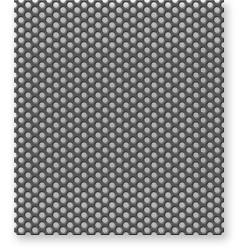# Resonant Sound Absorbers (Part V)

### Example 10:

Example #4 in Part One concerned a sheet of 16 gauge sheet metal, perforated with 1/8" holes, staggered on 3/8" centers, as a facing for a 3/4" glass fiber blanket against a solid wall. We found that the resonance frequency is 2000 Hz.

We now assume that the glass fiber material has a flow resistivity, 2 = 55 cgs rayls/in; accordingly, R = 3/4" x 55 = 41 cgs rayls, and R/pc = 1.

We first calculate the maximum sound absorption coefficient reached at the resonance frequency:

O.max = 1/[1/2 + (1/4)(R/pc + pc/R)] = 1/[1/2 + (1/4)(1 + 1) = 1.0.
Next we calculate the half-power bandwidth:

6fH = 2Tr[1 + (R/pc)](h/c)fR2
= 2Tr[1 + 1](0.75/13560)(2000)2 = 2794 Hz.

The lower and upper frequency bounds for the half-power bandwidth are:

l = fr -(6fH/2)
= 2000 - 1397 = 603Hz f2 = fR + (6fH/2)
= 2000 + 1397 = 3397 Hz

The value of the absorption coefficient at frequencies f1 and f2 is 0.50 (open triangles). The entire frequency plot of absorption for this example is given on Figure 27.

Figure 27. Frequency dependence of the absorption coefficients for the tuned resonant absorbers of Examples 10-13.